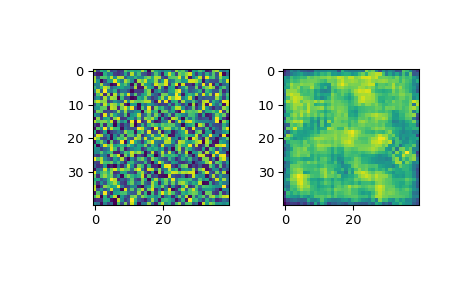# scipy.signal.wiener¶

scipy.signal.wiener(im, mysize=None, noise=None)[source]

Perform a Wiener filter on an N-dimensional array.

Apply a Wiener filter to the N-dimensional array im.

Parameters
imndarray

An N-dimensional array.

mysizeint or array_like, optional

A scalar or an N-length list giving the size of the Wiener filter window in each dimension. Elements of mysize should be odd. If mysize is a scalar, then this scalar is used as the size in each dimension.

noisefloat, optional

The noise-power to use. If None, then noise is estimated as the average of the local variance of the input.

Returns
outndarray

Wiener filtered result with the same shape as im.

Notes

This implementation is similar to wiener2 in Matlab/Octave. For more details see 

References

1

Lim, Jae S., Two-Dimensional Signal and Image Processing, Englewood Cliffs, NJ, Prentice Hall, 1990, p. 548.

Examples

```>>> from scipy.misc import face
>>> from scipy.signal.signaltools import wiener
>>> import matplotlib.pyplot as plt
>>> import numpy as np
>>> rng = np.random.default_rng()
>>> img = rng.random((40, 40))    #Create a random image
>>> filtered_img = wiener(img, (5, 5))  #Filter the image
>>> f, (plot1, plot2) = plt.subplots(1, 2)
>>> plot1.imshow(img)
>>> plot2.imshow(filtered_img)
>>> plt.show()
```